Home | ARTS | Operations Management | Determination of Project Completion Time in PERT

# Determination of Project Completion Time in PERT

Posted On :  23.06.2018 11:31 pm

Find out the time required to complete the following project and the critical activities:

Determination of Project Completion Time in PERT

Problem 2

Find out the time required to complete the following project and the critical activities: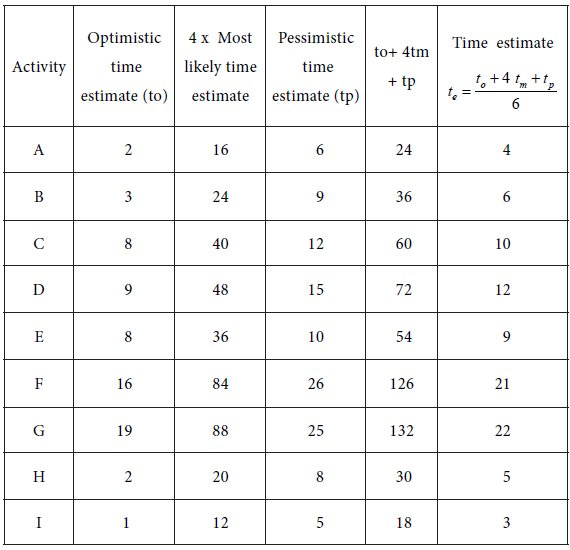Using the single time estimates of the activities, we get the following network diagram for the project.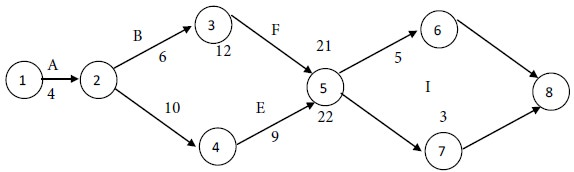Consider the paths, beginning with the start node and stopping with the end node. There are four such paths for the given project. They are as follows: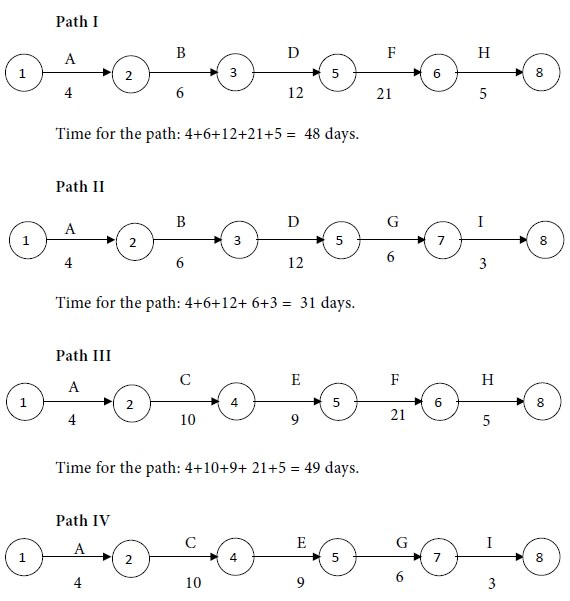Time for the path: 4+10+9+ 6+3 = 32 days.

Compare the times for the four paths.

Maximum of {48, 31, 49, 32} = 49.

We see that Path III has the maximum time.

Therefore the critical path is Path III. i.e.,The critical activities are A, C, E, F and H.

The non-critical activities are B, D, G and I.

Project time (Also called project length) = 49 days.

Problem 3

Find out the time, variance and standard deviation of the project with the following time estimates in weeks:Solution

From the three time estimates tp  , tm  and to  , calculate te  for each activity. We obtain the following table: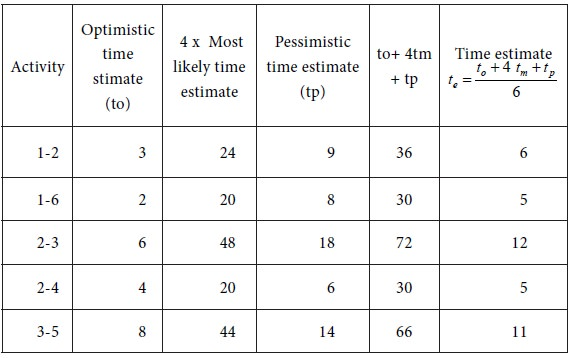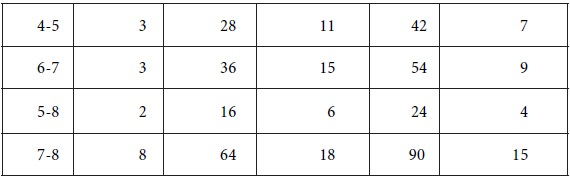With the single time estimates of the activities, we get the following network diagram for the project.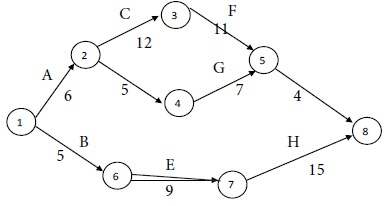Consider the paths, beginning with the start node and stopping with the end node. There are three such paths for the given project. They are as follows:## Calculation of Standard Deviation and Variance for the Critical Activities: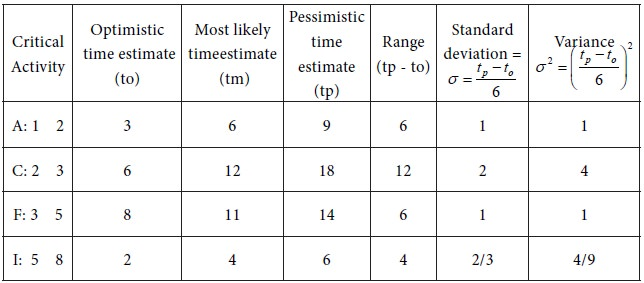Variance of project time (Also called Variance of project length) = Sum of the variances for the critical activities = 1+4+1+ 4/9 = 58/9 Weeks.
Standard deviation of project time = √Variance = √58/9 = 2.54 weeks.

Problem 4

A project consists of seven activities with the following time estimates. Find the probability that the project will be completed in 30 weeks or less.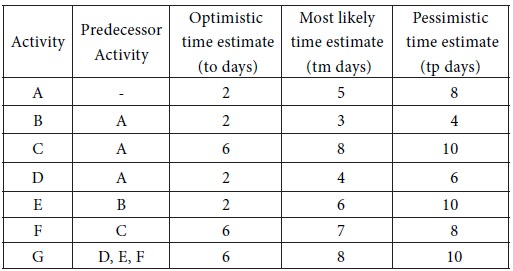Solution

From the three time estimates ,  and , calculate  for each activity. The results are furnished in the following table: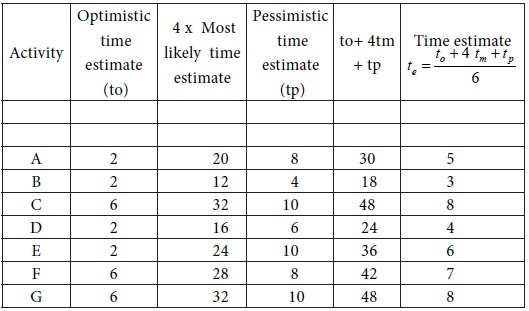With the single time estimates of the activities, the following network diagram is constructed for the project.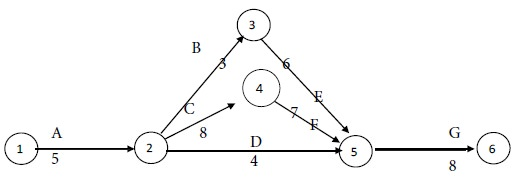Consider the paths, beginning with the start node and stopping with the end node. There are three such paths for the given project. They are as follows: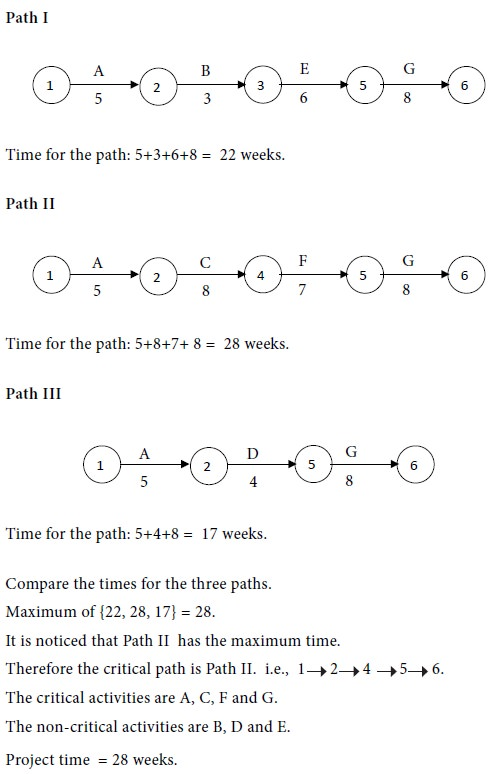## Calculation of Standard Deviation and Variance for the Critical Activities: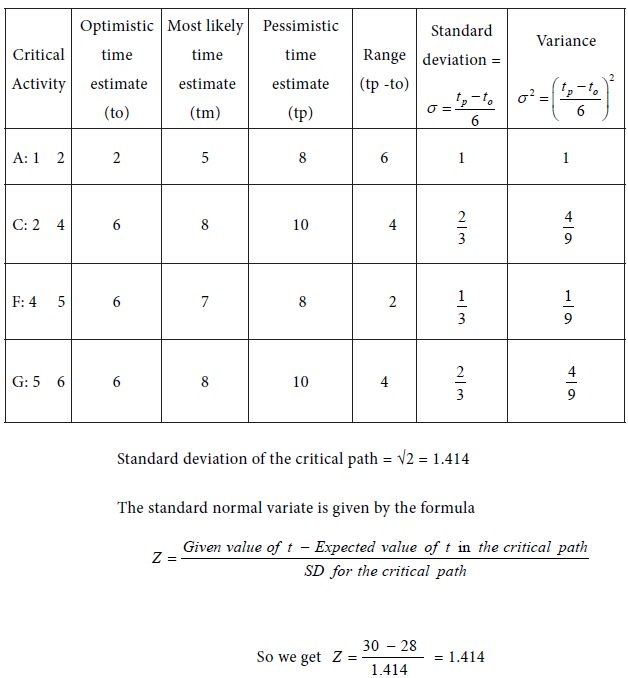We refer to the Normal Probability Distribution Table.

Corresponding to Z = 1.414, we obtain the value of 0.4207

We get 0.5 + 0.4207 = 0. 9207

Therefore the required probability is 0.92

i.e., There is 92% chance that the project will be completed before 30 weeks. In other words, the chance that it will be delayed beyond 30 weeks is 8%

Tags : Operations Management - Network Problems
Last 30 days 893 views